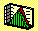2.7 SAMPLE STATISTICS: RELATIVE EFFICIENCY

The standard deviation in portfolio theory is used to measure the risk of a portfolio. In sample statistics, the standard deviation is used as a measure of efficiency when there is more than one statistic available to estimate the underlying population parameter from. For example, for a normally distributed random variable, both the mean and the median coincide. In a sample the two statistics are most likely different. The question regarding which is better motivates the definition of relative efficiency.

Let S and G be two statistics. The ratio of the standard deviations corresponding to S/G is a measure of the efficiency of S relative to G.

The relative efficiency for the two statistics, mean and median, depends upon the underlying population distribution, whether it is skewed or symmetrical. For example, for a normally distributed random variable, the sample mean provides a more efficient estimate than does the sample median.

In the next topic we introduce the notion of sufficient statistics.

previous topic

next topic